Courses

# Short Answer Type Questions(Part- 2)- Circles Class 10 Notes | EduRev

## Class 10 : Short Answer Type Questions(Part- 2)- Circles Class 10 Notes | EduRev

The document Short Answer Type Questions(Part- 2)- Circles Class 10 Notes | EduRev is a part of the Class 10 Course Class 10 Mathematics by VP Classes.
All you need of Class 10 at this link: Class 10

Q12. Two tangents PA and PB are drawn to a circle with centre O from an external point P. Prove  that ∠APB = 2∠OAB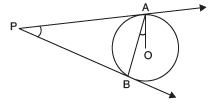Sol. We have PA and PB, the tangents to the circle and O is the centre of the circle.
∴ PA = PB
⇒∠2 = ∠4  ...(1)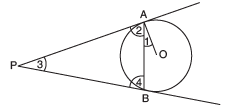Since the tangent is perpendicular to the radius through the point of contact,
∴∠OAP = 90°
⇒ ∠1 + ∠2 = 90° ...(2)
⇒ ∠2 = 90° − ∠1
Now, in ∆ABP, we have:
∴∠2 + ∠3 + ∠4 = 180°
⇒∠2 + ∠3 + ∠2 = 180° [From (1)]
⇒∠2 + ∠3 = 180°
⇒ 2 (90° − ∠1) + ∠3 = 180° [From (2)]
⇒ 180° − 2 ∠1 + ∠3 = 180°
⇒ 2 ∠1 = ∠3
⇒ ∠3 = 2∠1
⇒∠APB = 2∠OAB

Q13. ABC is an isosceles triangle, in which AB = AC, circumscribed about a circle. Show that BC is bisected at the point of contact.

Sol. We know that the tangents to a circle from an external point are equal.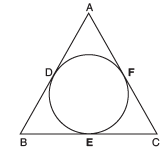Similarly,
BD = BE
and CE = CF
Since AB = AC [Given]
⇒ AB − AD = AC − AF [∵ AD = AF]
⇒ BD = CF ...(1)
But BF = BD and CF = CE
∴ From (1), we have:
BE = CE

Q14. If a, b, c are the sides of a right triangle where c is hypotenuse, prove that the radius r of the circle which touches the sides of the triangle is given by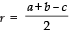Sol. Here, a, b and c are the sides of rt D ABC such that BC = a, CA = b and AB = c Let the circle touches the sides BC, CA, AB at D, E and F respectively.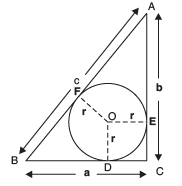= AE = AF and BD = BF
Also, CE = CD = r
∴ AF = b – r BF = a – r
Now, AB = c  ⇒  (AF + BF)
=(b – r) + (a – r)
⇒ c = b + a – 2r
⇒ 2r = a + b – cQ15. In a right Δ ABC, right angled at B, BC = 5 cm and AB = 12 cm. The circle is touching the sides of Δ ABC. Find the radius of the circle.

Sol. Let the circle with centre O and radius ‘r’ touches AB, BC and AC at P, Q, R, respectively.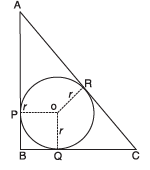Now,
AR = AP
∵ AP = AB – BP = (12 – r) cm
∴ AR = (12– r)cm
Similarly, CR = (5 – r)cm
Now, using Pythagoras theorem in rt Δ ABC, we have
AC2 = AB2 + BC2
⇒ AC2 = 122 + 52
⇒ AC = 13 cm
But AC = AR + CR = (12 – r) + (5 – r)
⇒ (12 – r) + (5 – r) = 13 cm
⇒ 17 – 2r = 13 cm
⇒ 2 r = 17 – 13 = 4 cm
⇒ r = 4/2 = 2 cm

Thus, the radius of the circle is 2 cm.

Q16. Prove that the parallelogram circumscribing a circle is a rhombus.

Sol. Since ABCD is a ║ gm
∴ AB = CD
∵ Tangents from an external point to a circle are equal,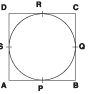⇒(AP + PB) + (RC + DR)
= (AS + DS) + (BQ + QC)
⇒ AB + CD = AD + BC
⇒ AB = AD = CD = BC
i.e., ABCD is a rhombus.

Q17. In the following figure, OP is equal to diameter of the circle. Prove that ABP is an equilateral triangle.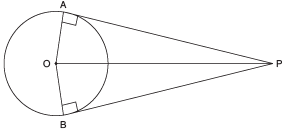Sol. Since the tangent is perpendicular to the radius through the point of contact,
∴ ∠OAP = 90°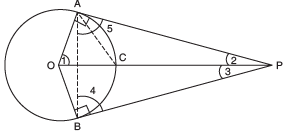Let us join AB and AC.
In right ∆OAP, OP is the hypotenuse and C is the mid point of OP.
[∵ OP is a diameter of the circle (given)]
∴ CA = CP = CO = Radius of the circle.
∴ ΔOAC is an equilateral triangle.
Since all angles in an equilateral triangle are 60°,
∴∠1 = 60°
Now, in ∆OAP, we have
∠1 + ∠OAP + ∠2 = 180°
⇒ 60° + 90° + ∠2 = 180°
⇒∠2 = 180° − 90° − 60° = 30°
Since PA and PB make equal angles with OP,
∴∠2= ∠3 ⇒∠3 = 30°
∴∠APB = ∠2 + ∠3
= 30° + 30° = 60°
Again, PA = PB.
⇒ In  ΔABP,∠4= ∠5
[Angles opposite to equal sides are equal]
Now, in ΔABP, ∠4 + ∠5 + ∠APB = 180°
⇒∠4 + ∠4 + ∠APB= 180°
⇒ 2∠4 + ∠60° = 180°
⇒ 2∠4 = 180° − 60° = 120°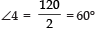Since, ∠4 = 60° ∠5 = 60°
∵ ΔABP is an equilateral Δ.
∠APB = 60°

Q18. Prove that the angle between the two tangents to a circle drawn from an external point is supplementary to the angle subtended by the line segment joining the points of contact at the centre.

Or

Two tangents PA and PB are drawn from an external point P to a circle with centre O. Prove that AOBP is a cyclic quadrilateral.

Sol.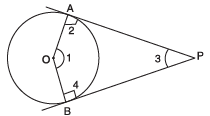We have tangents PA and PB to the circle from the external point P. Since a tangent to a circle is perpendicular to the radius through the point of contact,
∴ ∠2 = 90° and ∠4 = 90°
∠1 + ∠2 + ∠3 + ∠4 = 360°
⇒∠1 + 90° + ∠3 + 90° = 360°
⇒∠1 + ∠3 = 360° − 90° − 90°
= 180°
i.e., ∠1 and ∠3 are supplementary angles.
⇒∠ AOB and ∠APB are supplementary
⇒ AOBP is a cyclic quadrilateral.

Q19. Two equal circles, with centres O and O′, touch each other at X. OO′ produced meets the circle with centre O′ at A. AC is tangent to the circle with centre O, at the point C. O′D is perpendicular to AC. Find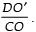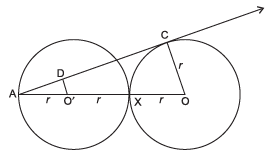Sol. AC is tangent to circle with centre O at C (given)
∠ACO = 90°  ⇒ ∆ ACO is a rt Δ
∠ADO′ = 90°   [ ä O′D ⊥ AC] ⇒ Δ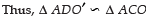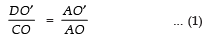But, AO′ = r,     O′X = r  and  OX = r ⇒ AO = 3r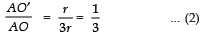From (1) and (2), we get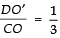Q20. Out of two concentric circles, the radius of the outer circle is 5 cm and the chord AC of length 8 cm is a tangent to the inner circle. Find the radius of the inner circle.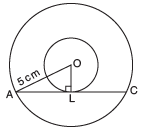Sol. Let the given chord AC of the larger circle touch the smaller circle at L. ∵ AC is a tangent at L to the smaller circle with centre O
∴ OL ⊥ AC
Also AC is a chord of the bigger circle

∴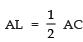Now, in rt. ΔOAL,
OL2 = OA2 – AL2

or OL2 = 52 – 42
= (5 + 4) (5 – 4)
= 9 × 1 = 9

⇒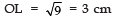Thus, the radius of the inner circle is 3 cm.

Q21. In the figure, O is the centre of a circle of radius 5cm. T is a point such that OT = 13cm and  OT intersects circle at E. If AB is a tangent to the circle at E, find the length of AB, where TP and TQ are two tangents to the circle.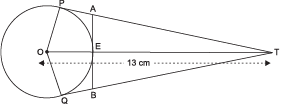Sol. O is centre of the circle and PT is a tangent to circle
∴ ∠ OPT = 90°  ⇒ ∆ OPT is a rt ∆ using Pythagoras theorem
OT2 = OP2 + PT2   or 132 = 52 + PT2
⇒ PT2 =132 – 52
⇒ PT =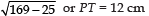Let AP = AE = x
[Tangent to a circle from an external point are equal]
⇒ AT = PT – AP = (12 – x) cm
[∵ AB is a tangent to the circle at E and OE is a radius]
∴ ∠ OEA = 90° ⇒ ∠AET  = 90°
Δ AET is a rt ∆
⇒ AT2 = AE2 + ET2
or (12 − x)2 = x2 + (13 − 5)2
⇒  144− 24x + x2 = x2 + 64 or  24x = 80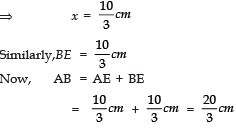Offer running on EduRev: Apply code STAYHOME200 to get INR 200 off on our premium plan EduRev Infinity!

132 docs

,

,

,

,

,

,

,

,

,

,

,

,

,

,

,

,

,

,

,

,

,

;